Publication date: 08/13/2020

## Control Limits for XBar and S Charts

JMP generates control limits for XBar and S charts as:

LCL for XBar chart =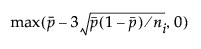UCL for XBar chart =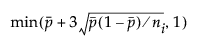LCL for S chart =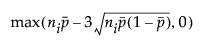UCL for S chart =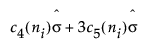Center line for S chart: By default, the center line for the ith subgroup (where 3 is the sigma multiplier) indicates an estimate of the expected value of si. This value is computed as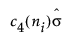, where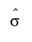is an estimate of σ.

The estimate for the standard deviation for XBar and S charts is: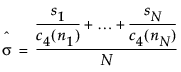where: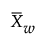= weighted average of subgroup means

σ = process standard deviation

ni = sample size of ith subgroup

c4(n) is the expected value of the standard deviation of n independent normally distributed variables with unit standard deviation

c5(n) is the standard deviation of the standard deviation of n independent normally distributed variables with unit standard deviation

N is the number of subgroups for which ni ≥ 2

si is the sample standard deviation of the ith subgroup